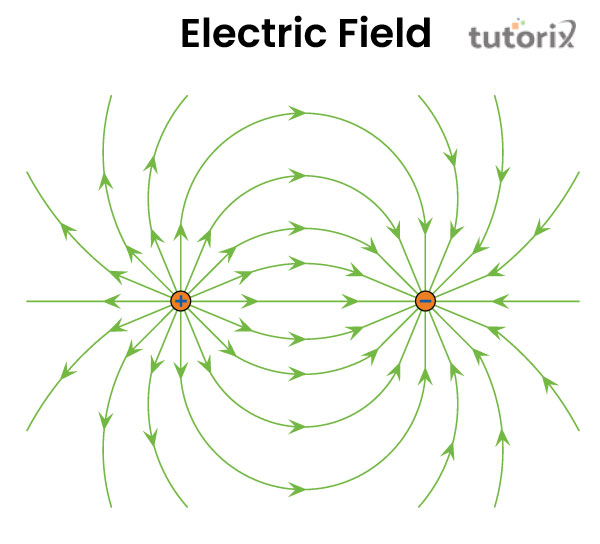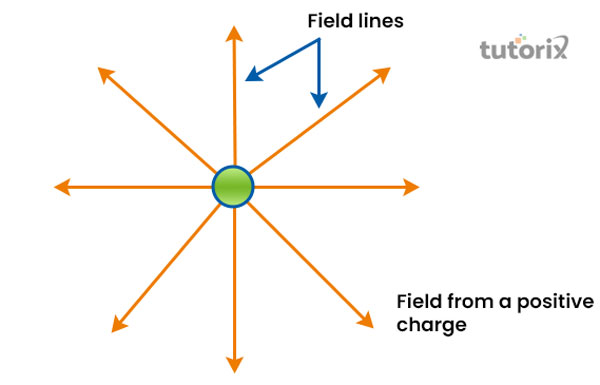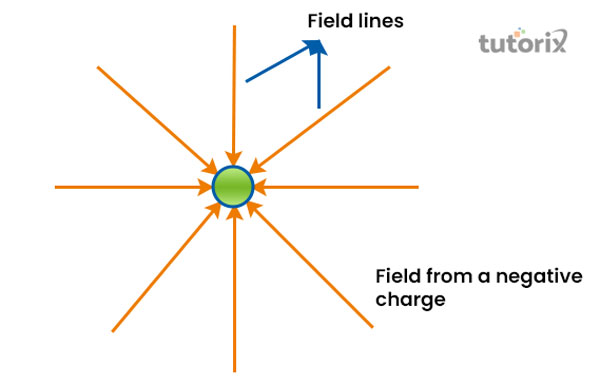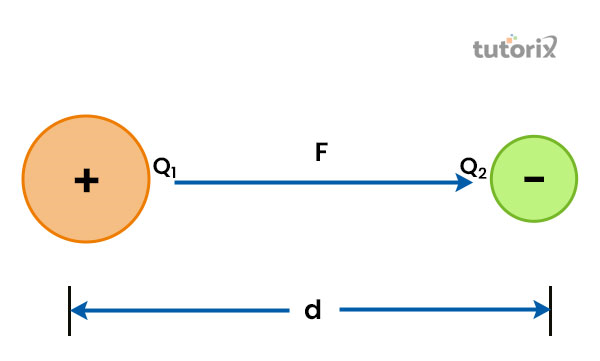# Physical significance of electric field

## Introduction

The electric field is an elegant way that helps in the characterization of an electrical environment within a particular system of charges. Here, a force per unit is defined with E, F and Q while applying this in electro-physics. It commonly tells about the environment that is formed around charges. It can be defined in different points of space and can be generally referred to the quantity that defines every point of space.

## What is Electric Field?

The electric field is an independent system property of test charges that is expressed by E in electro-physics. It is an elegant way for characterization of an electrical environment where a particular system of charge is present. The electric field is a point in space that represents a unit's force of the positive charges that can be experienced after a certain point of time in space.Figure 1: Electric field

An electric field refers to the quantity that defines different points in space which generally varies from one point to another (Electricaltechnology, 2022). It appears as a physical field around the charged particles within a field. An electric field can be spotted either in an attracting state or in a repelling state in a certain space. It also helps in the analysis of a force within a unit charge at any point in space (Namboodiri, 2018).

## Formula of Electric Field

An electric field is commonly produced by the electric charges within a space that is formed around a component that is being charged with electric force. It exists in almost all points of space where it can be observed by bringing other charges into the electric field. An electric field is commonly denoted by E, F and Q. Here, E represents the electric field, F represents the electric force and Q is the test charge present around an electric field (Benea-Chelmus et al. 2019). So, the general formula of the electric field appears as E = F/Q. Similarly, when charges appear far from one another, the value of the electric field also appears as 0. An electric field is a vector quantity that goes in the same as well as the opposite direction from the charges.

For example, F equals to $k\rvert q1q2\rvert/r2$ (Openstax.org, 2022). It defines that since q2 has been defined with test charges after the substitute is applied, it becomes F, $E=[K\rvert q_{1}\rvert]/r^{2}$

## Definition of Electric Charge

An electric charge is a property of matter that attracts or repels each other. The charge can be positive or negative based on the attractive or repel capability between matters. The opposite charge between matters can be positive to negative, positive to positive or negative to negative based on their capability of repelling.Figure 2: Positive electric charge

A positive electric charge involves more protons than electrons while involving positive and negative charges. When an object involves more protons, it can be identified as a positively charged object. In terms of positive charges, the field lines appear in the outward direction (Khan et al. 2018). For example, if a glass is rubbed with silk, it becomes positively charged, as there are more protons present in the glass than the number of electrons in the glass.Figure 3: Negative electric charge

A negative charge incorporates more electrons than protons while charging an object. When the object has more numbers of electrons than the number of protons present in the object, it can be defined as negatively charged (Sciencefacts.net, 2022). In terms of a negative charge, the field lines of an electric field are in the inward direction. For example, if a glass is rubbed with silk, the silk becomes negatively charged as it has more electrons than a proton while charging the object.

## Coulomb's Law

According to Coulomb's law, electric charges repel or attract each other, as forces are exerted on each other. Here, the force between different electric points is ideally charged and concentrated at a particular point in space. The law states that the magnitude or strength of the force is proportional to each other.Figure 4: Coulomb's Law

The experiment of Coulomb is based on the inverse square law and involves force between 2 different masses that are inversely proportional. The first law of Coulomb states that the positive charges repel each other whereas the negative charges attract each other (Electricaltechnology.org, 2022). The second law says that between the two charging bodies (Q1and Q2 here) the common relationship is directly proportional to each other when electric force (F) is applied properly.

## Physical Significance of an Electric Field

### Under Static Condition

Under the static condition, the electrical environment that surrounds a system of charges is charecterized by an electric field. At every point, the characterization can vary from one point to another (Namboodiri, 2018).

### Under Non-Static Electromagnetic Condition

Under this condition, the electromagnetic waves are raised by the accelerated motions while propagating with speed and imparting a certain force on the other charges. Here, the electric field and the magnetic field are associated with the transportation of energy. By nature, the interaction between the charges appears electromagnetic under this condition. Both the electric and magnetic fields are perceived as physical entities as both of them can be detected by their forces (Khan et al. 2018). In simple words, the physical significance of the electric fields can evolve according to their own laws under different circumstances.

## Conclusion

A certain amount of force is always present within an electric field. As force is a vector and it has a deep connection with an electric field, the field can also be defined as a vector field that moves in the same direction of the force. In simple words, an electric field is an independent system of charges that conveniently tells about the electrical environment around the charges. In addition, the characterization can vary from one point to another when propagated and imparted with a certain force.

## FAQs

Q1. Can an electric field be created without the charged particles?

Yes, an electromagnetic field can be created without the support of charged particles. It can involve so-called vacuum solutions from the Maxwell's equation to assess nontrivial solutions.

Q2. What are the attraction and repulsion of the Electric field lines?

In terms of attraction, the electric field lines are pointed towards the negative charges from the positive charges. Unlike attraction, the field lines travel from negative charges toward positive charges in terms of repelling.

Q3. What are some of the examples of the Electric field?

An electric field is generated when an electric lamp is connected to a power source through an electric connection. Another example is the transmission of radio or television signals from antennas. Here, an electric field is formed around the antenna while transmitting signals.

Q4. What is the SI unit for measuring the Electric field?

The electric field is measured with the SI unit of volts per meter (V/m). In addition, Newton and Coulomb are also used to measure a unit of charge within the electric fields.

## References

### Book

Namboodiri, C. K. (2018). Development of a glossary for the technical terms of the NCERT Physics text books at senior secondary level. Retrieved from: http://ir.riemysore.ac.in:8080/jspui/bitstream/123456789/122/1/Development%20of%20a%20glossary%20for%20the%20technical%20terms%20of%20the%20NCERT%20Physics%20text%20books%20at%20senior%20secondary%20level-2018.pdf

### Journals

Benea-Chelmus, I. C., Settembrini, F. F., Scalari, G., & Faist, J. (2019). Electric field correlation measurements on the electromagnetic vacuum state. Nature, 568(7751), 202-206. Retrieved from: https://arxiv.org/pdf/1809.01785

Khan, H., Haneef, M., Shah, Z., Islam, S., Khan, W., & Muhammad, S. (2018). The combined magneto hydrodynamic and electric field effect on an unsteady Maxwell nanofluid flow over a stretching surface under the influence of variable heat and thermal radiation. Applied Sciences, 8(2), 160. Retrieved from: https://www.mdpi.com/2076-3417/8/2/160/pdf

### Websites

Sciencefacts.net, (2022). Positive and Negative charge. Retrieved from: https://www.sciencefacts.net/electric-field.html [Retrieved on 2nd June, 2022]